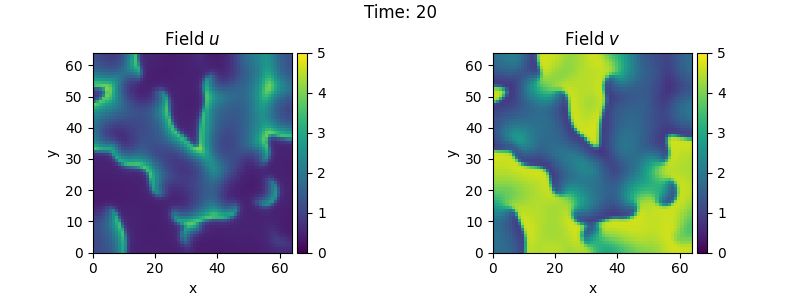# 2.26. Brusselator - Using custom class

This example implements the Brusselator with spatial coupling,

$\begin{split}\partial_t u &= D_0 \nabla^2 u + a - (1 + b) u + v u^2 \\ \partial_t v &= D_1 \nabla^2 v + b u - v u^2\end{split}$

Here, $$D_0$$ and $$D_1$$ are the respective diffusivity and the parameters $$a$$ and $$b$$ are related to reaction rates.

Note that the PDE can also be implemented using the PDE class; see the example. However, that implementation is less flexible and might be more difficult to extend later./home/docs/checkouts/readthedocs.org/user_builds/py-pde/checkouts/0.30.0/pde/grids/boundaries/local.py:1822: NumbaDeprecationWarning: The 'nopython' keyword argument was not supplied to the 'numba.jit' decorator. The implicit default value for this argument is currently False, but it will be changed to True in Numba 0.59.0. See https://numba.readthedocs.io/en/stable/reference/deprecation.html#deprecation-of-object-mode-fall-back-behaviour-when-using-jit for details.
def virtual_point(
/home/docs/checkouts/readthedocs.org/user_builds/py-pde/checkouts/0.30.0/examples/pde_brusselator_class.py:59: NumbaDeprecationWarning: The 'nopython' keyword argument was not supplied to the 'numba.jit' decorator. The implicit default value for this argument is currently False, but it will be changed to True in Numba 0.59.0. See https://numba.readthedocs.io/en/stable/reference/deprecation.html#deprecation-of-object-mode-fall-back-behaviour-when-using-jit for details.
def pde_rhs(state_data, t):


import numba as nb
import numpy as np

from pde import FieldCollection, PDEBase, PlotTracker, ScalarField, UnitGrid

class BrusselatorPDE(PDEBase):
"""Brusselator with diffusive mobility"""

def __init__(self, a=1, b=3, diffusivity=[1, 0.1], bc="auto_periodic_neumann"):
super().__init__()
self.a = a
self.b = b
self.diffusivity = diffusivity  # spatial mobility
self.bc = bc  # boundary condition

def get_initial_state(self, grid):
"""prepare a useful initial state"""
u = ScalarField(grid, self.a, label="Field $u$")
v = self.b / self.a + 0.1 * ScalarField.random_normal(grid, label="Field $v$")
return FieldCollection([u, v])

def evolution_rate(self, state, t=0):
"""pure python implementation of the PDE"""
u, v = state
rhs = state.copy()
d0, d1 = self.diffusivity
rhs = d0 * u.laplace(self.bc) + self.a - (self.b + 1) * u + u**2 * v
rhs = d1 * v.laplace(self.bc) + self.b * u - u**2 * v
return rhs

def _make_pde_rhs_numba(self, state):
"""nunmba-compiled implementation of the PDE"""
d0, d1 = self.diffusivity
a, b = self.a, self.b
laplace = state.grid.make_operator("laplace", bc=self.bc)

@nb.jit
def pde_rhs(state_data, t):
u = state_data
v = state_data

rate = np.empty_like(state_data)
rate = d0 * laplace(u) + a - (1 + b) * u + v * u**2
rate = d1 * laplace(v) + b * u - v * u**2
return rate

return pde_rhs

# initialize state
grid = UnitGrid([64, 64])
eq = BrusselatorPDE(diffusivity=[1, 0.1])
state = eq.get_initial_state(grid)

# simulate the pde
tracker = PlotTracker(interval=1, plot_args={"vmin": 0, "vmax": 5})
sol = eq.solve(state, t_range=20, dt=1e-3, tracker=tracker)


Total running time of the script: ( 0 minutes 13.192 seconds)